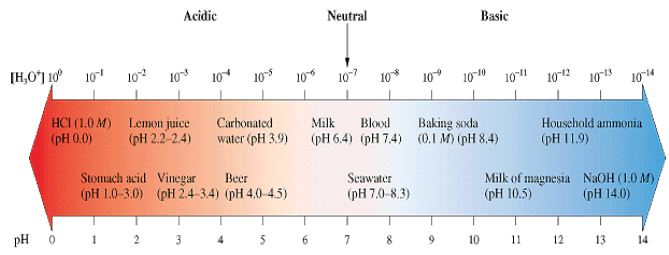# Solutions of pH

Although one can quantitatively describe the acidity of a solution by its concentration [H+], it is more convenient to determine the acidity in terms of pH. The pH of a solution is defined as the negative logarithm of the molar hydrogen ion concentration as follows:

pH = -log10 [H+]

Example

For a solution in which the hydrogen-ion concentration is 1.0 x 10-3, the pH is:

pH = -log10 (1.0 x 10-3) = 3.00

Remember that the number of decimal places in the pH equals the number of significant figures in the hydrogen-ion concentration. Additionally, pH has no units.

pH of solutions

In a neutral solution, the hydrogen-ion concentration is 1.0 x 10-7 M, therefore pH is equal to 7.00. For acidic solutions, the hydrogen-ion concentration is greater than 1.0 x 10-7 M, so the pH is less than 7.00. Similarly, a basic solution has a pH greater than 7.00. Remember that a strong acid has a low pH.

The pH Scale

In the Figure below are shown the pH values of some common solutions.